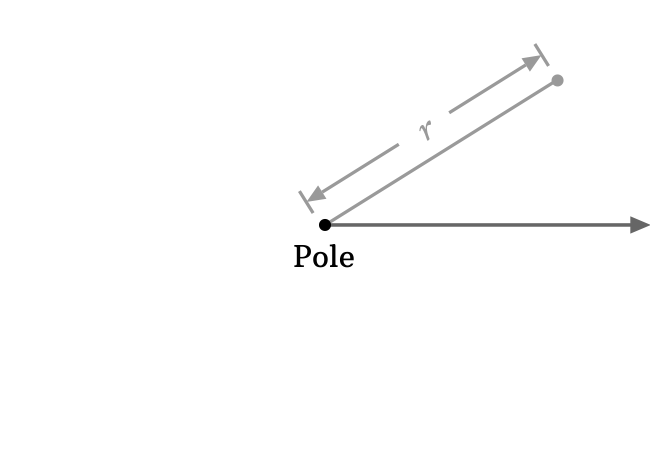# Pole

A point from which the distance of any point is measured on the two-dimensional plane is called the pole.

## IntroductionThere are lots of points on a two-dimensional plane and it is very difficult to identify the position of every point. So, a particular point should be considered as a reference point and from which, the distance of every point on the plane is measured easily.

The point is called the pole of the polar coordinate system. The pole is exactly a center (or centre) point of the two-dimensional plane.

### Example

Let’s learn the concept of a pole in the polar coordinate system from understandable animation.Let $P$ and $Q$ be two points on a two dimensional plane. They both are not maintaining equal distance in all four directions. So, either of them cannot be used as a reference point to identify the location of any point in the plane.

For this reason, let’s consider a point, which is exactly middle to the plane in all four directions and it is denoted by $O$ and a ray is drawn in the right direction horizontally to express an axis.

The point $O$ is called a pole in the polar coordinate system and it is used as a reference point to measure the distance of any point. Thus, the pole used to identify the location of every point. In this example, the points $P$ and $Q$ are located at distances of a and b respectively.

Latest Math Topics
Jun 26, 2023
Jun 23, 2023

###### Math Questions

The math problems with solutions to learn how to solve a problem.

Learn solutions

Practice now

###### Math Videos

The math videos tutorials with visual graphics to learn every concept.

Watch now

###### Subscribe us

Get the latest math updates from the Math Doubts by subscribing us.